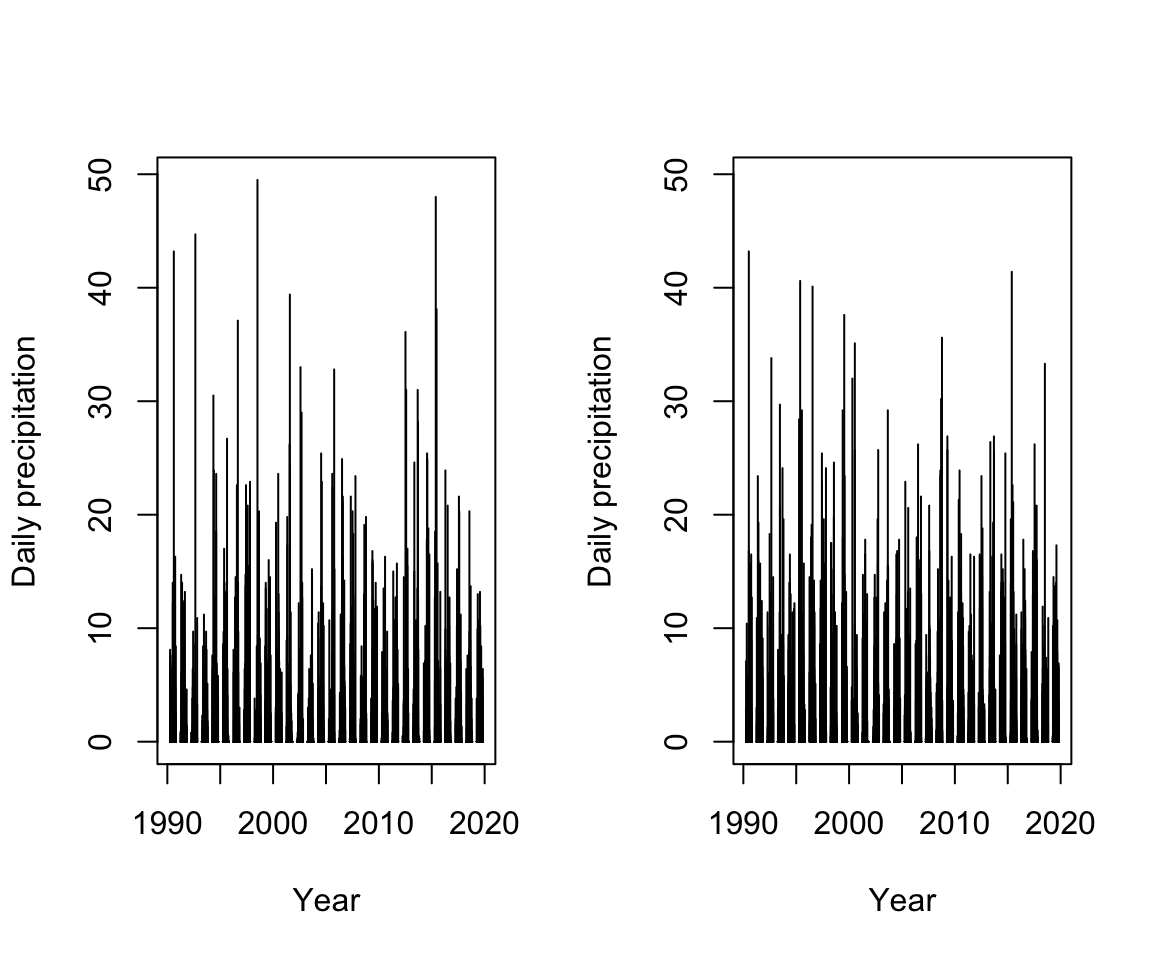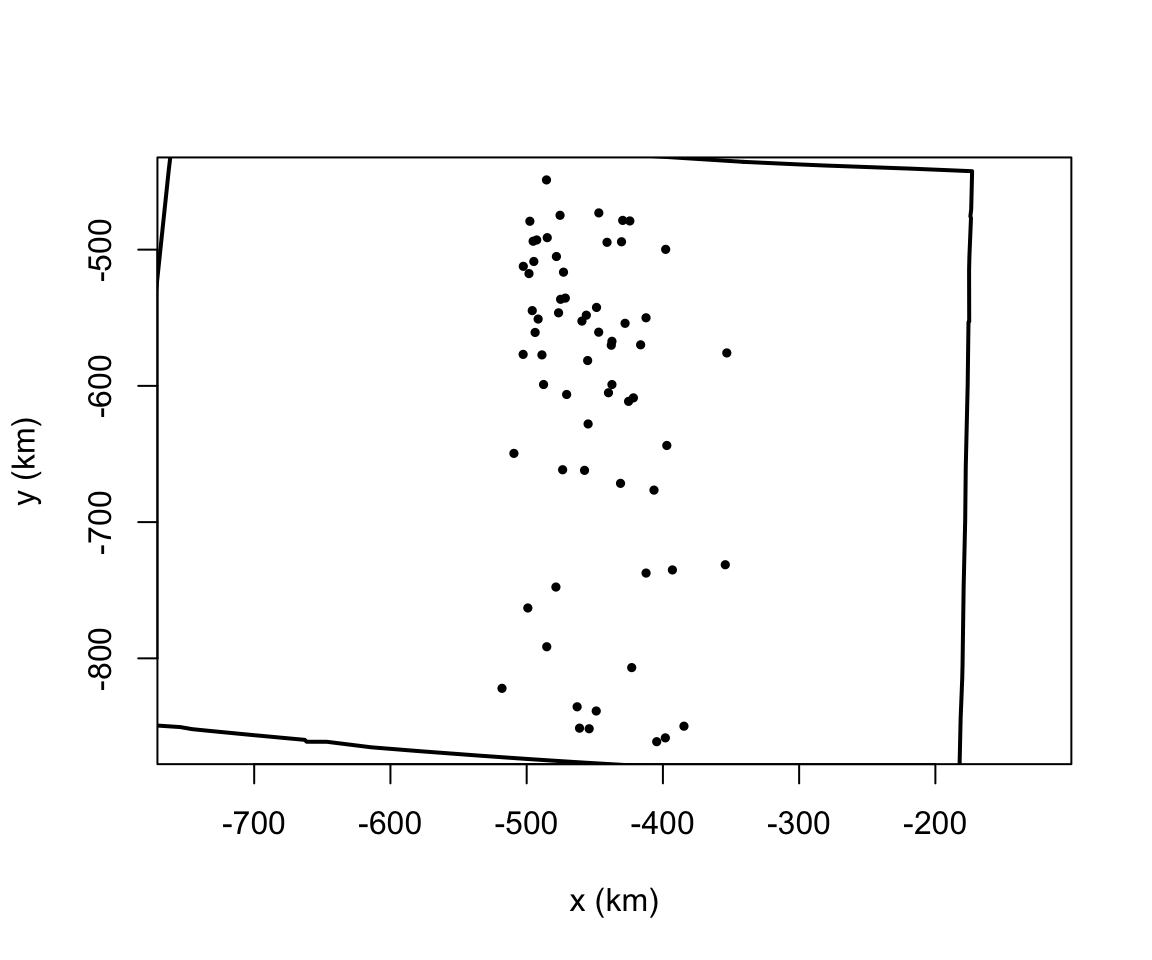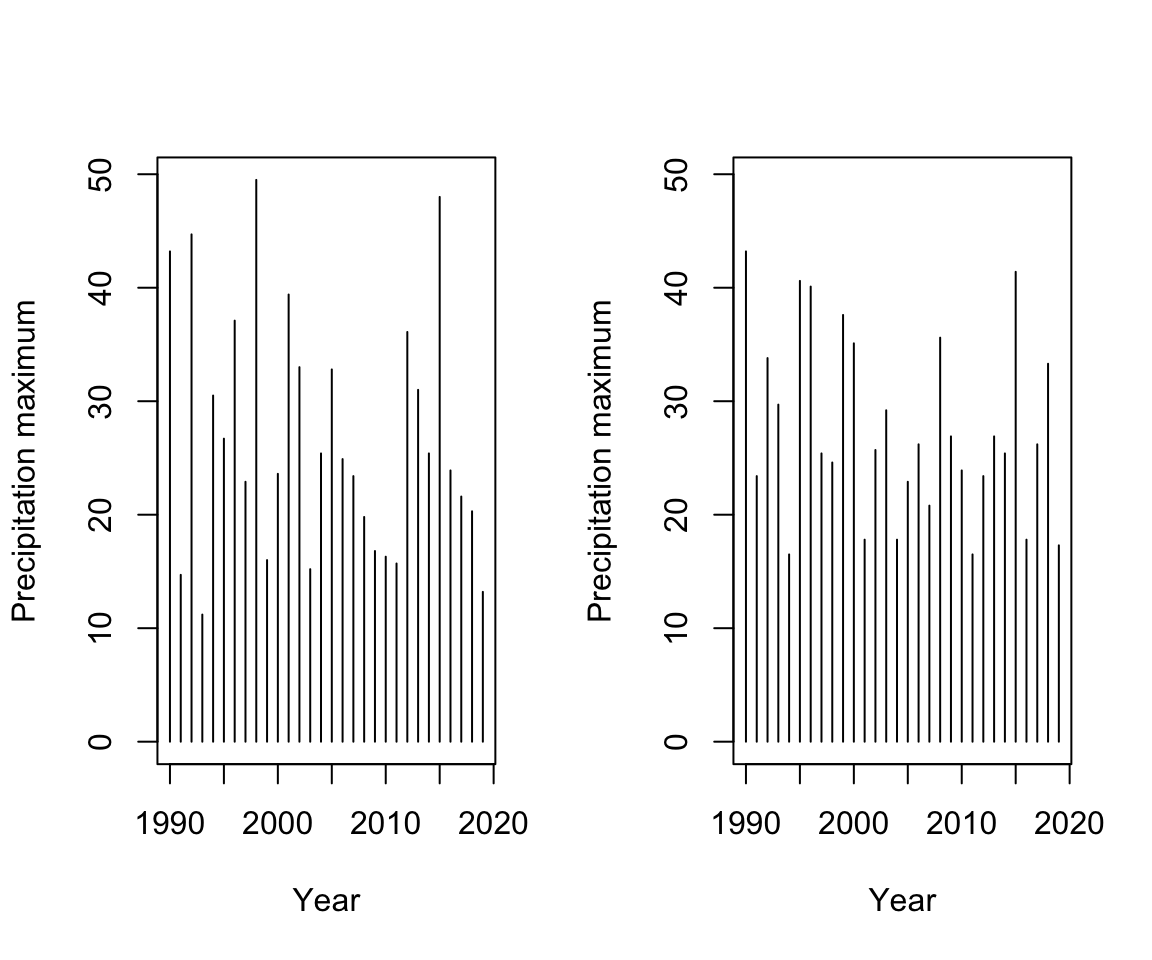# 1 Introduction

This tutorial focuses on extreme-value modeling using the GAMs and the Integrated Nested Laplace Approximation (INLA) implemented in the INLA package, which is usually referred to as “R-INLA”. We first load a few libraries that will be necessary. If R-INLA is not yet installed, please uncomment the corresponding line and run the relevant `install.packages()` command.

``````# install.packages('INLA',repos=c(getOption('repos'),INLA='https://inla.r-inla-download.org/R/stable'),
# dep=TRUE)
library(evgam)  # frequentist GAMs, and Colorado precipitation dataset
library(extRemes)  # for univariate extremes
library(fields)  #for plotting (image.plot)
# library(geostatsp) # library for geostatistical modeling making use of
# INLA
library(grDevices)  # for color palettes
library(INLA)  # implementation of INLA
# library(inlabru) # high-level interface to R-INLA with additional modeling
# tools
library(maps)  # mapping tools
library(sp)  # spatial tools
library(viridis)``````

In the evgam package, we have a data frame with observations of daily precipitation amounts at 64 gauge stations in the state of Colorado, US, during the summer months of April to October (type “?COprcp” for more information about this dataset).

``````data(COprcp)
dim(COprcp)``````
``##  404326      3``
``head(COprcp)``
``````##         date prcp meta_row
## 1 1990-04-01  0.0        1
## 2 1990-04-02  0.5        1
## 3 1990-04-03  0.0        1
## 4 1990-04-04  0.0        1
## 5 1990-04-05  0.0        1
## 6 1990-04-06  8.1        1``````
``str(COprcp)``
``````## 'data.frame':    404326 obs. of  3 variables:
##  \$ date    : Date, format: "1990-04-01" "1990-04-02" ...
##  \$ prcp    : num  0 0.5 0 0 0 8.1 0 0 0 0.8 ...
##  \$ meta_row: int  1 1 1 1 1 1 1 1 1 1 ...``````
``head(COprcp_meta)``
``````##            id            name       lon     lat   elev
## 1 USC00050263     ANTERO RSVR -105.8919 38.9933 2718.8
## 2 USC00050454          BAILEY -105.4764 39.4053 2362.8
## 3 USC00050848         BOULDER -105.2667 39.9919 1671.5
## 4 USC00050950   BRIGHTON 3 SE -104.8361 39.9436 1528.9
## 5 USC00051060 BUCKHORN MTN 1E -105.2969 40.6158 2255.5
## 6 USC00051179     BYERS 5 ENE -104.1275 39.7406 1554.8``````
``````n_sites = nrow(COprcp_meta)
coor = cbind(COprcp_meta\$lon, COprcp_meta\$lat)
dates = as.POSIXlt(COprcp\$date)
dates_unique = sort(unique(dates))
# beginning of observation period:
``````##  "1990-04-01 UTC" "1990-04-02 UTC" "1990-04-03 UTC" "1990-04-04 UTC"
##  "1990-04-05 UTC" "1990-04-06 UTC"``````
``````# end of observation period:
tail(dates_unique)``````
``````##  "2019-10-26 UTC" "2019-10-27 UTC" "2019-10-28 UTC" "2019-10-29 UTC"
##  "2019-10-30 UTC" "2019-10-31 UTC"``````
``````n_days = length(unique(dates))
years = 1900 + dates\$year
range(years)``````
``##  1990 2019``
``````months = 1 + dates\$mon  # month (1-12)
ydays = 1 + dates\$yday  # day within year (1-366)
obs = COprcp\$prcp
id_coor = COprcp\$meta_row
obs_mat = matrix(NA, nrow = n_days, ncol = n_sites)
# fill the matrix:
for (i in 1:n_sites) {
rows_i = which(id_coor == i)
obs_mat[match(dates[rows_i], dates_unique), i] = obs[rows_i]
}
# Are there any missing values?
mean(is.na(obs_mat))  # yes, around 1.6% of all values are missing. ``````
``##  0.01595113``
``````# Show the time series for two locations:
par(mfrow = c(1, 2))
plot(dates_unique, obs_mat[, 1], type = "h", ylim = c(0, max(obs_mat[, c(1,
20)], na.rm = TRUE)), xlab = "Year", ylab = "Daily precipitation")
plot(dates_unique, obs_mat[, 20], type = "h", ylim = c(0, max(obs_mat[, c(1,
20)], na.rm = TRUE)), xlab = "Year", ylab = "Daily precipitation")````````````# export as pdf: dev.copy(pdf, file = 'precipitation-series.pdf', width =
# 12) dev.off()``````

For potential later use, we transform longitude-latitude to a coordinate system that has been optimized for the United States and corresponds to metric distances for the study region. This is recommendable for accurate modeling of the spatial correlations since the metric distance of one unit latitude is (slightly) different from one unit longitude. We use the EPSG codes for projections (European Petroleum Survey Group):

• 4326 for longitude-latitude;
• 2163 for US National Atlas Equal Area.

For the spatial distance unit, we use 1km. Note that the some implementations may sometimes show stability problems with very large coordinate values (e.g. expressed in meters).

``````coor_sp = SpatialPoints(coords = coor, proj4string = CRS("+init=epsg:4326"))
coor_metric = spTransform(coor_sp, CRS("+init=epsg:2163"))@coords/10^3
# We prepare the boundary coordinates of the state of Colorado:
mymap = map("state", regions = "colorado", plot = FALSE)
# Transform coordinates (those that are not 'NA') to EPSG 2163 (in km unit)
# using spTransform:
xy_region = SpatialPoints(coords = na.omit(cbind(mymap\$x, mymap\$y)), proj4string = CRS("+init=epsg:4326"))
xy_region = spTransform(xy_region, CRS("+init=epsg:2163"))@coords  # in m unit
xy_region = xy_region/10^3  # in km unit
mymap\$x[!is.na(mymap\$x)] = xy_region[, 1]
mymap\$y[!is.na(mymap\$y)] = xy_region[, 2]
# We plot observation sites and the Colorado boundary:
plot(coor_metric, pch = 19, cex = 0.5, asp = 1, xlab = "x (km)", ylab = "y (km)")
lines(mymap\$x, mymap\$y, lwd = 2)``````# 3 Part I: Univariate extreme-value theory

## 3.1 Annual maxima

For modeling annual maxima, we generate a data matrix containing only the annual maxima, where rows correspond to years and columns correspond to observation locations. We use the aggregate-function to extract annual maxima (where we remove missing data during a year). The result of the aggregate-function is a data frame with first column indicating the year, and the other columns reporting the annual maxima. We transform this data frame to numerical matrix containing only the maxima values. For illustration, we plot the series of annual maxima for two observation locations.

``````maxima = aggregate(obs_mat, by = list(year = 1900 + dates_unique\$year), FUN = max,
na.rm = TRUE)  # first column of maxima contains the year
maxima = as.matrix(maxima[, -1])
maxima[maxima == -Inf] = NA
par(mfrow = c(1, 2))
plot(unique(1900 + dates_unique\$year), maxima[, 1], type = "h", ylim = c(0,
max(maxima[, 1:2])), xlab = "Year", ylab = "Precipitation maximum")
plot(unique(1900 + dates_unique\$year), maxima[, 20], type = "h", ylim = c(0,
max(maxima[, 1:2])), xlab = "Year", ylab = "Precipitation maximum")``````For a (very) simple GEVD model, we could estimate GEVD parameters for the entire sample of maxima. For this purpose, we here use the extRemes package. Several other packages implement maximum likelihood estimation of the GEV and GPD (e.g. packages evd, fExtremes).

``````max_sample = as.numeric(maxima)
max_sample = na.omit(max_sample)
fit = fevd(max_sample, type = "GEV", method = "MLE", period.basis = "year",
use.phi = TRUE)
summary(fit)``````
``````##
## fevd(x = max_sample, use.phi = TRUE, type = "GEV", method = "MLE",
##     period.basis = "year")
##
##  "Estimation Method used: MLE"
##
##
##  Negative Log-Likelihood Value:  7671.267
##
##
##  Estimated parameters:
##   location      scale      shape
## 27.7792569 10.6739940  0.1021712
##
##  Standard Error Estimates:
##   location      scale      shape
## 0.27200262 0.20443419 0.01583819
##
##  Estimated parameter covariance matrix.
##              location         scale         shape
## location  0.073985426  0.0261594943 -0.0012695922
## scale     0.026159494  0.0417933383 -0.0003801474
## shape    -0.001269592 -0.0003801474  0.0002508484
##
##  AIC = 15348.53
##
##  BIC = 15365.21``````
``# plot(fit) # visualizations to check goodness-of-fit``

We obtain a slightly positive value of the shape parameter (xi), which is typical for precipitation data.

## 3.2 Threshold exceedances

For a (very) simple Peaks-over-Threshold model of precipitation data, we could choose a global threshold as a high quantile of positive precipitation intensities, for instance at the 95% level. After fixing the threshold, we can explore the resulting exceedances (how many?, histogram, etc.).

``````prob = 0.95
thresh = quantile(obs_mat[obs_mat > 0], prob, na.rm = TRUE)
sum(as.numeric(obs_mat > thresh), na.rm = TRUE)``````
``##  5894``
``hist((obs_mat - thresh)[obs_mat > thresh], xlab = "Excesses", main = "Histogram of excesses")``